# A shopkeeper

A shopkeeper cuts a wheel of cheese into 10 equal wedges. A customer buys one-fifth of the wheel. How many wedges does the customer buy? Use the number line to help find the solution.

n =  2

### Step-by-step explanation: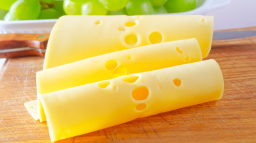Did you find an error or inaccuracy? Feel free to write us. Thank you!Tips to related online calculators
Need help to calculate sum, simplify or multiply fractions? Try our fraction calculator.

## Related math problems and questions:

• Diamonds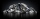If simva had 8 diamond and cuts them each into 1/3 pieces, then how many pieces does she have?
• A shopkeeper 2A shopkeeper charged E17 for 4 textbooks of the same kind. What is the cost of one textbook?
• Wire cut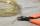A wire of length 9 m was cut into equal lengths using 5 cuts. How long is each piece?
• Unknown numberFind the unknown number equal to a quarter of a fifth of a number, which is by 152 more than an unknown number.
• Dairy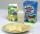Diary workers calculated according to the standards from the 108 litres of milk is possible to produce 9 kg cheese. How many tons of cheese was possible according to standards make from milk from 100 cows devoted for 30 days with average daily milk yield
• A shopkeeper 3A shopkeeper sells 8 1/3 kg, 10 1/4 kg and 11 1/5 kg of apples on 3 consecutive days. What is the total weight of apples sold?
• Cheaper cars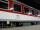State-owned railway company buys 10 air-conditioned coaches for nearly 18 million euros. Calculate how many euros is the equivalent of one seat in the wagon with a capacity of 83 people. How many of used cars at the price 2700 Euros can be buy instead ofWhy does 1 3/4 + 2 9/10 equal 4.65? How do you solve this?
• MilimetersHow many millimeters are 1/4 meters?
• Milk2cheese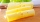From 40 liters of milk is produced 8 kg of cheese how many liters of milk are needed to produce 2 kg of cheese?
• Golf ballsOf the 28 golf balls, 1/7 are yellow. How many golf balls are yellow? Use the model to help you. Enter your answer in the box.
• Woodworking classKeith is making a spice rack for his mom in woodworking class. He starts with a board that is 31.25 inches long. Then, he cuts it into 5 equal pieces for the shelves. How long will each shelf be?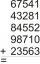Fill letters instead of digits so the indicated sum (equal letters represent equal digits). What number is hidden under the letter J? A A H A H O A H O J -------------------------- 4 3 2 1Ski organizers should print the start numbers from 1 to 45. How many times will they use the number 3 when printing?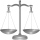A supermarket has 3 7/8 pounds of tangerines. Kelsey buys 1/2 of all the tangerines in the supermarket. How many pounds of tangerines did Kelsey buy?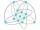The lesson of physical education, pupils are first divided into three groups so that each has the same number. The they redistributed, but into six groups. And again, it was the same number of children in each group. Finally they divided into nine equal gMs. Sheppard cuts ½ of a piece of construction paper. She uses ⅙ of the piece to make a flower. What fraction of the sheet of paper does she use to make the flower?